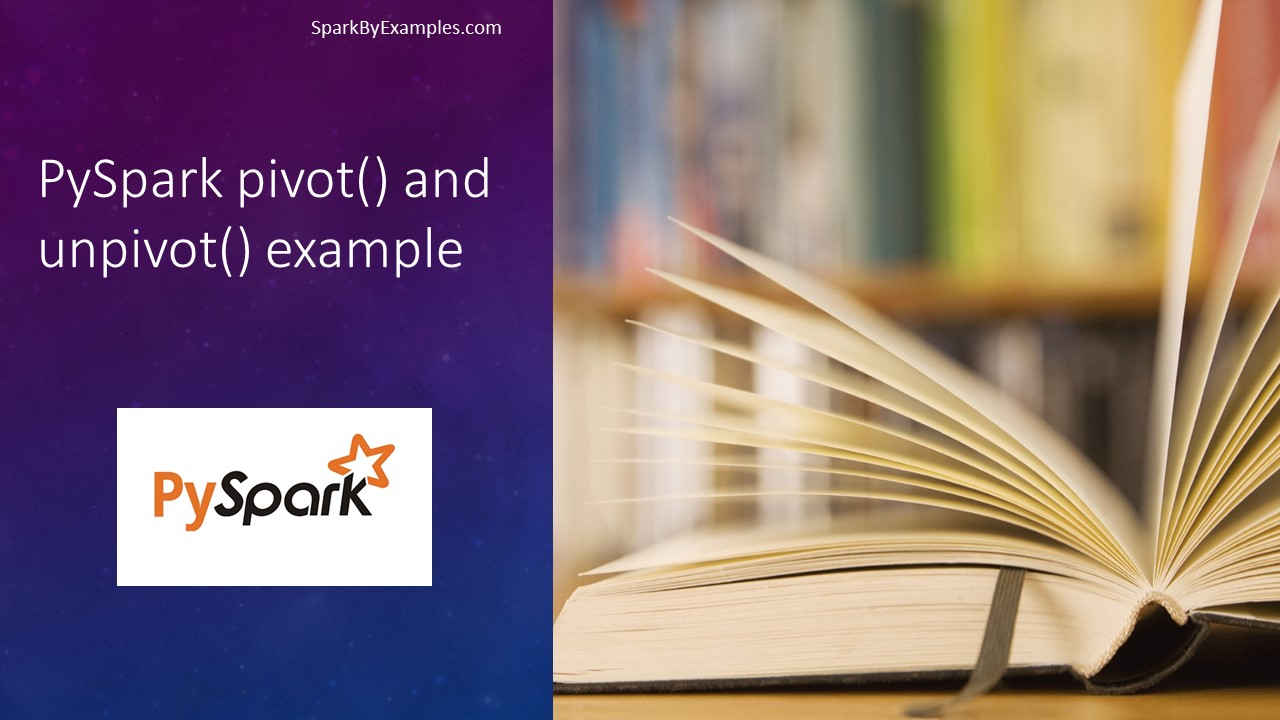# PySpark Pivot and Unpivot DataFrame

PySpark `pivot()` function is used to rotate/transpose the data from one column into multiple Dataframe columns and back using unpivot(). Pivot() It is an aggregation where one of the grouping columns values is transposed into individual columns with distinct data.

This tutorial describes and provides a PySpark example on how to create a Pivot table on DataFrame and Unpivot back.

Let’s create a PySpark DataFrame to work with.

``````
import pyspark
from pyspark.sql import SparkSession
from pyspark.sql.functions import expr
#Create spark session
data = [("Banana",1000,"USA"), ("Carrots",1500,"USA"), ("Beans",1600,"USA"), \
("Orange",2000,"USA"),("Orange",2000,"USA"),("Banana",400,"China"), \
("Carrots",1200,"China"),("Beans",1500,"China"),("Orange",4000,"China"), \

columns= ["Product","Amount","Country"]
df = spark.createDataFrame(data = data, schema = columns)
df.printSchema()
df.show(truncate=False)
``````

DataFrame ‘df’ consists of 3 columns `Product`, `Amount`, and `Country` as shown below.

``````
root
|-- Product: string (nullable = true)
|-- Amount: long (nullable = true)
|-- Country: string (nullable = true)

+-------+------+-------+
|Product|Amount|Country|
+-------+------+-------+
|Banana |1000  |USA    |
|Carrots|1500  |USA    |
|Beans  |1600  |USA    |
|Orange |2000  |USA    |
|Orange |2000  |USA    |
|Banana |400   |China  |
|Carrots|1200  |China  |
|Beans  |1500  |China  |
|Orange |4000  |China  |
|Beans  |2000  |Mexico |
+-------+------+-------+
``````

## Pivot PySpark DataFrame

PySpark SQL provides `pivot()` function to rotate the data from one column into multiple columns. It is an aggregation where one of the grouping columns values is transposed into individual columns with distinct data. To get the total amount exported to each country of each product, will do group by `Product`, pivot by `Country`, and the sum of `Amount`.

``````
pivotDF = df.groupBy("Product").pivot("Country").sum("Amount")
pivotDF.printSchema()
pivotDF.show(truncate=False)
``````

This will transpose the countries from DataFrame rows into columns and produces the below output. where ever data is not present, it represents as `null` by default.

``````
root
|-- Product: string (nullable = true)
|-- Canada: long (nullable = true)
|-- China: long (nullable = true)
|-- Mexico: long (nullable = true)
|-- USA: long (nullable = true)

+-------+------+-----+------+----+
+-------+------+-----+------+----+
|Orange |null  |4000 |null  |4000|
|Beans  |null  |1500 |2000  |1600|
|Banana |2000  |400  |null  |1000|
|Carrots|2000  |1200 |null  |1500|
+-------+------+-----+------+----+
``````

## Pivot Performance improvement in PySpark 2.0

version 2.0 on-wards performance has been improved on Pivot, however, if you are using the lower version; note that pivot is a very expensive operation hence, it is recommended to provide column data (if known) as an argument to function as shown below.

``````
pivotDF = df.groupBy("Product").pivot("Country", countries).sum("Amount")
pivotDF.show(truncate=False)
``````

Another approach is to do two-phase aggregation. PySpark 2.0 uses this implementation in order to improve the performance Spark-13749

``````
pivotDF = df.groupBy("Product","Country") \
.sum("Amount") \
.groupBy("Product") \
.pivot("Country") \
.sum("sum(Amount)") \
pivotDF.show(truncate=False)
``````

The above two examples return the same output but with better performance.

## Unpivot PySpark DataFrame

Unpivot is a reverse operation, we can achieve by rotating column values into rows values. PySpark SQL doesn’t have unpivot function hence will use the `stack()` function. Below code converts column countries to row.

``````
from pyspark.sql.functions import expr
unPivotDF = pivotDF.select("Product", expr(unpivotExpr)) \
.where("Total is not null")
unPivotDF.show(truncate=False)
unPivotDF.show()``````

It converts pivoted column “country” to rows.

``````
+-------+-------+-----+
|Product|Country|Total|
+-------+-------+-----+
| Orange|  China| 4000|
|  Beans|  China| 1500|
|  Beans| Mexico| 2000|
| Banana|  China|  400|
|Carrots|  China| 1200|
+-------+-------+-----+
``````

## Transpose or Pivot without aggregation

Can we do PySpark DataFrame transpose or pivot without aggregation?

Off course you can, but unfortunately, you can’t achieve using the Pivot function. However, pivoting or transposing the DataFrame structure without aggregation from rows to columns and columns to rows can be easily done using PySpark and Scala hack. please refer to this example.

## Complete Example

``````
import pyspark
from pyspark.sql import SparkSession
from pyspark.sql.functions import expr
spark = SparkSession.builder.appName('SparkByExamples.com').getOrCreate()

data = [("Banana",1000,"USA"), ("Carrots",1500,"USA"), ("Beans",1600,"USA"), \
("Orange",2000,"USA"),("Orange",2000,"USA"),("Banana",400,"China"), \
("Carrots",1200,"China"),("Beans",1500,"China"),("Orange",4000,"China"), \

columns= ["Product","Amount","Country"]
df = spark.createDataFrame(data = data, schema = columns)
df.printSchema()
df.show(truncate=False)

pivotDF = df.groupBy("Product").pivot("Country").sum("Amount")
pivotDF.printSchema()
pivotDF.show(truncate=False)

pivotDF = df.groupBy("Product","Country") \
.sum("Amount") \
.groupBy("Product") \
.pivot("Country") \
.sum("sum(Amount)")
pivotDF.printSchema()
pivotDF.show(truncate=False)

""" unpivot """
unPivotDF = pivotDF.select("Product", expr(unpivotExpr)) \
.where("Total is not null")
unPivotDF.show(truncate=False)
``````

#### Conclusion:

We have seen how to Pivot DataFrame with PySpark example and Unpivot it back using SQL functions. And also have seen how PySpark 2.0 changes have improved performance by doing two-phase aggregation.

Happy Learning !!

### Naveen (NNK)

Naveen (NNK) is a Data Engineer with 20+ years of experience in transforming data into actionable insights. Over the years, He has honed his expertise in designing, implementing, and maintaining data pipelines with frameworks like Apache Spark, PySpark, Pandas, R, Hive and Machine Learning. Naveen journey in the field of data engineering has been a continuous learning, innovation, and a strong commitment to data integrity. In this blog, he shares his experiences with the data as he come across. Follow Naveen @ @ LinkedIn